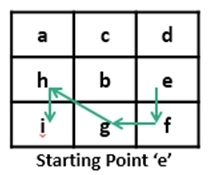# Longest consecutive path from a given starting character

A matrix of different characters is given. Starting from one character we have to find the longest path by traversing all characters which are greater than the current character. The characters are consecutive to each other.To find the longest path, we will use the Depth First Search algorithm. During DFS, some subproblems may arise multiple times. To avoid the computation of that, again and again, we will use a dynamic programming approach.

## Input and Output

Input:
The matrix as shown above. And the starting point. Here the starting point is e.
Output:
Enter Starting Point (a-i): e
Maximum consecutive path: 5

## Algorithm

findLongestLen(i, j, prev)

Input: Position i and j and the previous character.

Output: Longest length.

Begin
if (i, j) place is valid or prev and matrix[i,j] are adjacent, then
return 0
if longestPath[i, j] is already filled, then
return longestPath[i, j]
len := 0

for all its nearest 8 rooms k, do
len := maximum of len and (1 + findLongestLen(i, x[k], j +y[k], matrix[i, j]))
done

longestPath[i, j] := len
return len
End

getLen(start)

Input − Start point.

Output − Maximum length.

Begin
for all row r of matrix, do
for all column c, of matrix, do
if matrix[i, j] = start, then
for all adjacent room k, do
len := maximum of len and (1 + findLongestLen(i, x[k], j +y[k], matrix[i, j])))
done
done
done
return len
End

## Example

#include<iostream>
#define ROW 3
#define COL 3
using namespace std;

// tool matrices to recur for adjacent cells.
int x[] = {0, 1, 1, -1, 1, 0, -1, -1};
int y[] = {1, 0, 1, 1, -1, -1, 0, -1};
int longestPath[ROW][COL];

char mat[ROW][COL] = {
{'a','c','d'},
{'h','b','a'},
{'i','g','f'}
};

int max(int a, int b) {
return (a>b)?a:b;
}

bool isvalid(int i, int j) {
if (i < 0 || j < 0 || i >= ROW || j >= COL)    //when i and j are in range
return false;
return true;
}

bool isadjacent(char previous, char current) {
return ((current - previous) == 1);    //check current and previous are adjacent or not
}

int findLongestLen(int i, int j, char prev) {
return 0;

if (longestPath[i][j] != -1)
return longestPath[i][j];     //subproblems are solved already

int len = 0;  // Initialize result to 0

for (int k=0; k<8; k++)    //find length of the largest path recursively
len = max(len, 1 + findLongestLen(i + x[k], j + y[k], mat[i][j]));
return longestPath[i][j] = len;    // save the length and return
}

int getLen(char start) {
for(int i = 0; i<ROW; i++)
for(int j = 0; j<COL; j++)
longestPath[i][j] = -1;    //set all elements to -1

int len = 0;

for (int i=0; i<ROW; i++) {
for (int j=0; j<COL; j++) {    // check for all possible starting point
if (mat[i][j] == start)  {
for (int k=0; k<8; k++)    //for all eight adjacent cells
len = max(len, 1 + findLongestLen(i + x[k], j + y[k], start));
}
}
}
return len;
}

int main() {
char start;
cout << "Enter Starting Point (a-i): "; cin >> start;
cout << "Maximum consecutive path: " << getLen(start);
return 0;
}

## Output

Enter Starting Point (a-i): e
Maximum consecutive path: 5